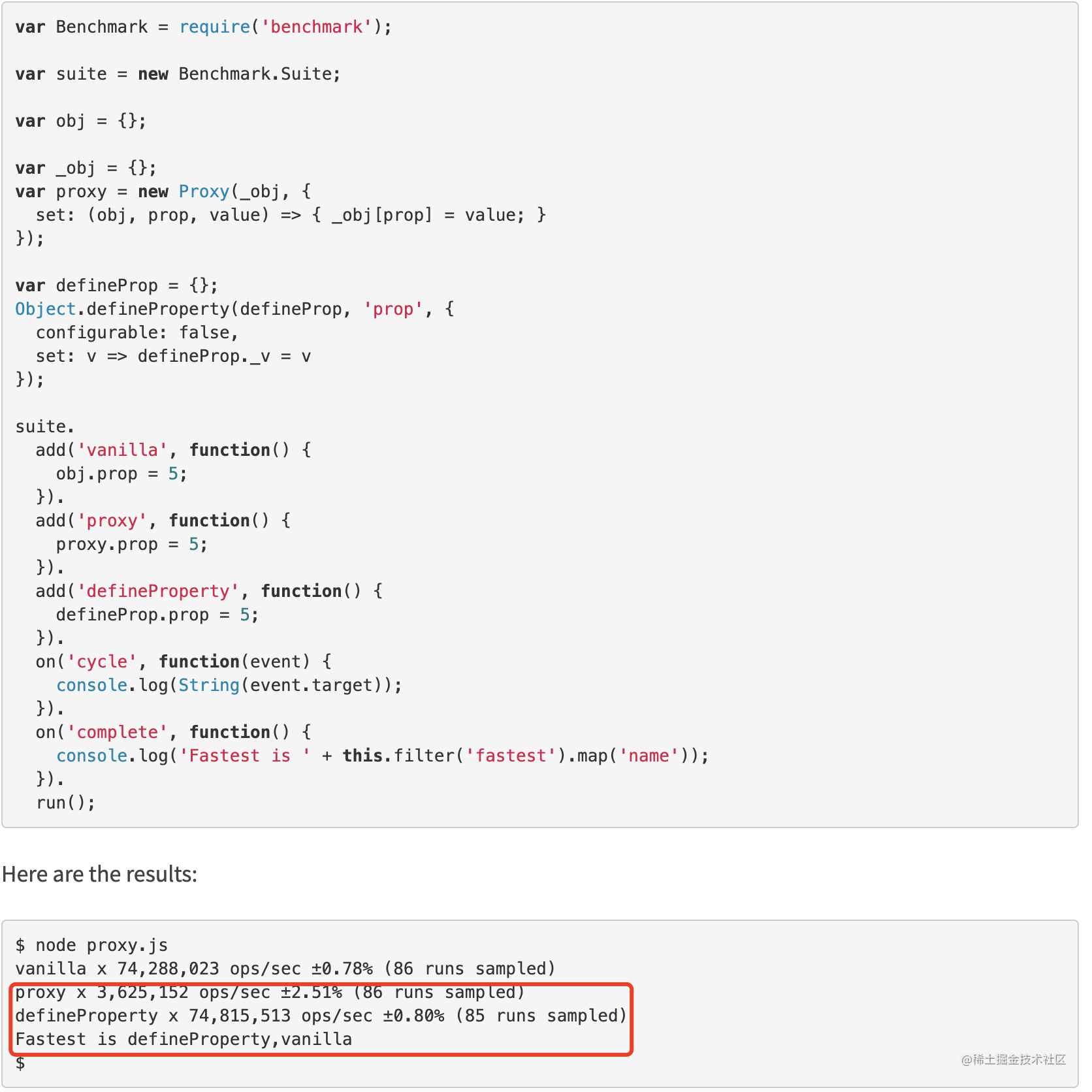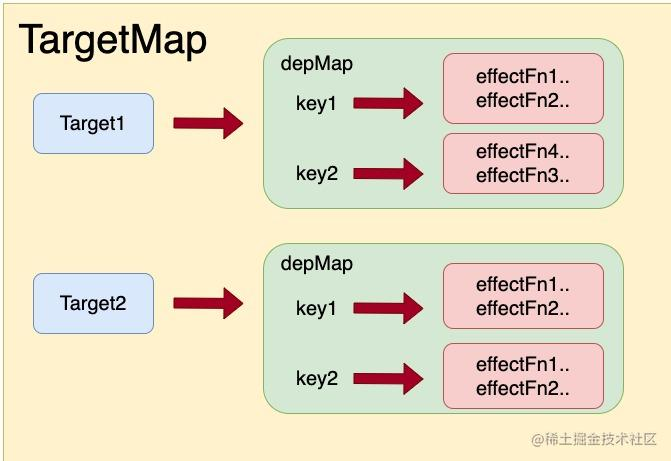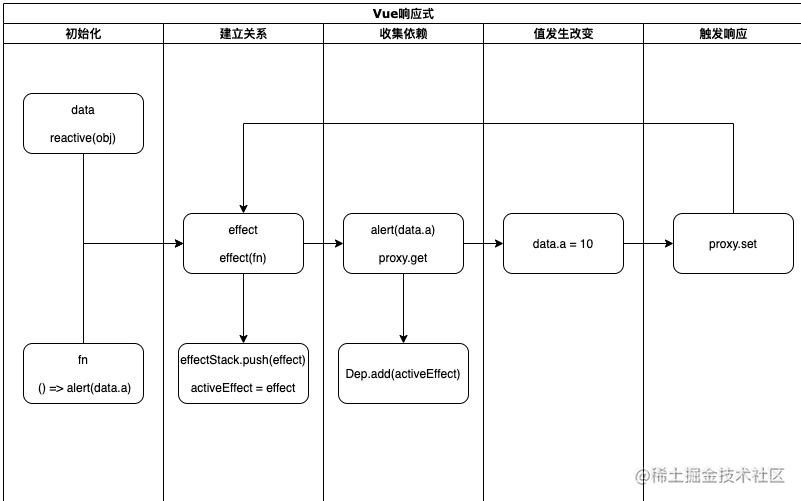Vue3.2中reactivity的优化__Vue.js__性能优化

# 前言

• More efficient ref implementation (~260% faster read / ~50% faster write)
• ~40% faster dependency tracking
• ~17% less memory usage

1. `ref` API 的读效率提升 `260%`，写效率提升约为 `50%` 。
2. 依赖收集的效率提升 `40%`
3. 内存占用减少 `17%` 。

# 一、Proxy

Proxy 对象用于创建一个对象的代理，从而实现基本操作的拦截和自定义（如属性查找、赋值、枚举、函数调用等）

## 1、 用法

new Proxy(target, handler)

``````const origin = {}
const proxy = new Proxy(origin, {
get(target, key, reciver) {
console.log('飒！')
return target[key]
},
set(target, key, value) {
target[key] = value
return true
}
})
proxy.a = 'aaaa'
proxy.a		// print 飒！
``````

## 2、Proxy并不快

Vue3.0使用了`proxy`可能会让人有错觉，`proxy`的速度比原本的`defineProperty`要快，其实并不是。这篇文章写了一些例子，比较详细的对比了`proxy``defineProperty`的执行速度。1. 在vue2.x中，受限于`Object.defineProperty`，收集依赖只能通过`getter`，触发依赖只能通过`setter`。新增属性，删除属性都无法触发响应式。
2. 同样的原因，我们无法让`Map、Set`这类数据类型转变为响应式，`Proxy`可以。
3. Vue2.x中，为了性能的考量，`数组通过劫持原生方法的方式实现的响应式`，但是通过`Proxy`我们不在去考虑数组的空位导致的empty问题。

## 3、Proxy并不是深层代理

``````const origin = {
data: {
a: 1,
b: 2
},
msg: 'message'
}

const handler = {
get(target, key, reciver) {
console.log('代理')
return Reflect.get(target, key, reciver)
}
}

const proxy = new Proxy(origin, handler)

proxy.msg // print 代理
const data = proxy.data // print 代理

data.a // 没有任何console.log
``````

Vue中`reactive`的实现，其实是递归的将对象全部都代理了一遍

# 二、Vue3.0的响应式

1. effect
2. effectFunction （简称fn）
3. Dep
4. ReactiveData

## 2、Dep## 3、effect

``````import { reactive, effect } from '@vue/reactivity'
const person = reactive({
name: 'Itachi',
age: 26
})

const fn = () => console.log(person.age)

effect(fn)
// print 26

person.age = 27
// print 27

``````

1. effectStack：栈用来保存当前正在执行的`effect`，因为`effect`之间经常会存在层叠的调用。
2. activeEffect：当前正在执行的栈顶的那个`effect`，好让`ReactiveData`更加精准的收集到这个依赖。

## 4、流程## 5、 源码

``````// 原始数据和proxy数据的映射
const proxyMap = new WeakMap()
// 依赖统一管理 { target => key => dep }
const targetMap = new WeakMap()
// effect执行栈
const effectStack = []
// 栈顶
const activeEffect = null

function reactive(target) {
// 如果已经reactive过，直接拿缓存
if (proxyMap.has(target)) return proxyMap.get(target)
// 简单类型无法proxy，直接返回值即可
if (typeof target !== 'object') return target

const proxy = new Proxy(target, {
get,
set
})
proxy
return proxy
}

function get(target, key, reciver) {
const res = Reflect.get(target, key, reciver)
// 收集
track(target, key)
if (isObject(res)) {
// 递归proxy
res = reactive(res)
}
return res
}

function set(target, key, value, reciver) {
const oldValue = traget[key]
const res = Reflect.set(target, key, value, reciver)
// 触发
trigger(target, key, value, oldValue)
return res
}

// 收集
function track(target, key) {
// deps的统一管理
let depsMap = targetMap.get(target)
if (!depsMap) {
targetMap.set(target, (depsMap = new Map()))
}
let dep = depsMap.get(key)
if (!dep) {
depsMap.set(key, (dep = new Set()))
}
// 收集依赖
if (dep.has(activeEffect)) {
activeEffect.deps.push(dep)
}
}

// 触发
function trigger(traget, key, value, oldValue) {
if (value === oldValue) return
let depsMap = tragetMap.get(target)
if (!depsMap) return
let deps = depsMap.get(key)
if (!deps) return
deps.forEach(effect => effect())
}

function effect(fn) {
const effect = function () {
// 清理上一次的缓存
cleanup(effect)
try {
effectStack.push(effect)
activeEffect = effect
return fn()
} finally {
effectStack.pop()
activeEffect = effectStack[effectStack.length - 1] || null
}
}
effect.raw = fn
effect.deps = []
return effect
}

// 重置依赖
function cleanup(effect) {
const { deps } = effect
for (let dep of deps) dep.delete(effect)
deps.length = 0
}

``````

# 三、位掩码

`｜、&、 ～ 、<<、>>` 这些位运算符

``````const ELEM = 1
const SVG = 1 << 2
const COMPONENT = 1 << 3
const FRAGMENG = 1 << 4
const PORTAL = 1 << 5

function isElement(vnode) {
return vnode.type & ELEM > 0
}
``````

# 四、Vue3.2是如何优化的

``````improt { reactive, effect } from '@vue/reactivity'

const data = reactive({ a: 1 })
effect(() => {  // effectTrackDepth = 0  trackOpbit = 1 << 0

console.log(data.a) // data => 'a' => dep.tag |= trackOpbit        dep.tag = 1

effect(() => {  // effectTrackDepth = 1  trackOpbit = 1 << 1

console.log(data.a + 1) // data => 'a' => dep.tag |= trackOpbit  dep.tag = 3

})
})

// 最后我们可以通过 dep.tag & 2 > 0  来判断该dep是否在特定的effect中使用过
``````

## 1、 改造Dep

Dep我们仅仅需要给原本的Set增加两个属性即可

``````// effect层级
const effectTrackDepth = 0
// 位标记
const trackOpBit = 1

// 收集
function track(target, key) {
// deps的统一管理
let depsMap = targetMap.get(target)
if (!depsMap) {
targetMap.set(target, (depsMap = new Map()))
}
let dep = depsMap.get(key)
if (!dep) {
depsMap.set(key, (dep = createDep()))
}
// 收集依赖
let shouldTrack = false
if (!newTracked(dep)) {
// 打上新标记
dep.n |= trackOpBit
shouldTrack = !wasTrack(dep) // 原本没有
}
if (shouldTrack) {
activeEffect.deps.push(dep)
}
}

// 创建Dep
function createDep() {
const dep = new Set()
dep.w = 0 // 旧标记
dep.n = 0 // 新标记
return dep
}
// 判断原来是否标记过
function wasTracked(dep) {
return (dep.w & trackOpBit) > 0
}
// 判断本次是否标记过
function newTracked(dep) {
return (dep.n & trackOpBit) > 0
}

``````

## 2、 改造effect

Effect我们需要做几个事情

1. 在effect执行前，先将effectTrackDepth++
2. 将原本收集到的dep打上自己的标记，作为旧标记
3. 执行期间通过track给dep.n打上新标记
4. 执行结束开始对比dep.w 和 dep.n，整理依赖
5. effectTrackDepth–

ok 我们来看代码

``````// 判断原来是否标记过
function wasTracked(dep) {
return (dep.w & trackOpBit) > 0
}
// 判断本次是否标记过
function newTracked() {
return (dep.n & trackOpBit) > 0
}
function initDepMarkers({ deps }) {
deps.forEach(dep => (dep.w |= trackOpBit))
}
function finalizeDepMarkers(effect) {
const { deps } = effect
if (deps.length) {
let ptr = 0
for (let dep of deps) {
if (wasTrack(dep) && !newTrack(dep)) {
// 之前收集到了这次没有
dep.delete(effect)
} else {
deps[ptr++] = dep
}
// 重置，为了下一次执行做准备
deps.w &= ~trackOpBit
deps.n &= ~trackOpBit
}
deps.length = ptr
}
}

function effect(fn) {
const effect = function () {
try {
// 标记effect层级
trackOpBit = 1 << ++effectTrackDepth
// 给之前收集到的依赖打上旧标记
initDepMarkers(effect)
effectStack.push((activeEffect = effect))
return fn()
} finally {
// 执行完effect，看一下需要删除那些依赖添加哪些依赖
finalizeDepMarkers(effect)
trackOpBit = 1 << --effectTrackDepth
effectStack.pop()
activeEffect = effectStack[effectStack.length - 1] || null
}
}
effect.raw = fn
effect.deps = []
return effect
}

``````

## 3、整体代码

``````// 原始数据和proxy数据的映射
const proxyMap = new WeakMap()
// 依赖统一管理 { target => key => dep }
const targetMap = new WeakMap()
// effect执行栈
const effectStack = []
// 栈顶
const activeEffect = null
// effect层级
const effectTrackDepth = 0
// 位标记
const trackOpBit = 1

function reactive(target) {
// 如果已经reactive过，直接拿缓存
if (proxyMap.has(target)) return proxyMap.get(target)
// 简单类型无法proxy，直接返回值即可
if (typeof target !== 'object') return target

const proxy = new Proxy(target, {
get,
set
})
proxy
return proxy
}

function get(target, key, reciver) {
const res = Reflect.get(target, key, reciver)
// 收集
track(target, key)
if (isObject(res)) {
// 递归proxy
res = reactive(res)
}
return res
}

function set(target, key, value, reciver) {
const oldValue = traget[key]
const res = Reflect.set(target, key, value, reciver)
// 触发
trigger(target, key, value, oldValue)
return res
}

// 收集
function track(target, key) {
// deps的统一管理
let depsMap = targetMap.get(target)
if (!depsMap) {
targetMap.set(target, (depsMap = new Map()))
}
let dep = depsMap.get(key)
if (!dep) {
depsMap.set(key, (dep = createDep()))
}
// 收集依赖
let shouldTrack = false
if (!newTracked(dep)) {
// 打上新标记
dep.n |= trackOpBit
shouldTrack = !wasTrack(dep) // 原本没有
}
if (shouldTrack) {
activeEffect.deps.push(dep)
}
}

// 触发
function trigger(traget, key, value, oldValue) {
if (value === oldValue) return
let depsMap = tragetMap.get(target)
if (!depsMap) return
let deps = depsMap.get(key)
if (!deps) return
deps.forEach(effect => effect())
}

// 创建Dep
function createDep() {
const dep = new Set()
dep.w = 0 // 旧标记
dep.n = 0 // 新标记
return dep
}
// 判断原来是否标记过
function wasTracked(dep) {
return (dep.w & trackOpBit) > 0
}
// 判断本次是否标记过
function newTracked() {
return (dep.n & trackOpBit) > 0
}
function initDepMarkers({ deps }) {
deps.forEach(dep => (dep.w |= trackOpBit))
}
function finalizeDepMarkers(effect) {
const { deps } = effect
if (deps.length) {
let ptr = 0
for (let dep of deps) {
if (wasTrack(dep) && !newTrack(dep)) {
// 之前收集到了这次没有
dep.delete(effect)
} else {
deps[ptr++] = dep
}
// 重置，为了下一次执行做准备
deps.w &= ~trackOpBit
deps.n &= ~trackOpBit
}
deps.length = ptr
}
}

function effect(fn) {
const effect = function () {
try {
// 标记effect层级
trackOpBit = 1 << ++effectTrackDepth
// 给之前收集到的依赖打上旧标记
initDepMarkers(effect)
effectStack.push((activeEffect = effect))
return fn()
} finally {
// 执行完effect，看一下需要删除那些依赖添加哪些依赖
finalizeDepMarkers(effect)
trackOpBit = 1 << --effectTrackDepth
effectStack.pop()
activeEffect = effectStack[effectStack.length - 1] || null
}
}
effect.raw = fn
effect.deps = []
return effect
}

``````